Short articles on topics in systematic trading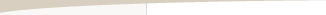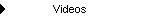Other Articles...Equity Curve Trading

by Michael R. Bryant

A money management technique that can sometimes improve trading performance is to modify the position sizing based on crossovers of a moving average of the equity curve. The basic idea is to either trade more or fewer contracts when the equity curve crosses above or below its moving average.

There are at least two basic ways to implement this idea. One is to stop trading when the equity curve crosses above or below its moving average and to resume trading on a crossover in the opposite direction. This is the most basic and commonly used method. You would typically stop trading when the equity curve crosses below the moving average if your system or method tends to produce streaks of wins and losses, so that when it starts to lose, it's best to stop trading until it starts winning again.

On the other hand, if your system or method tends to "revert to the mean" -- after several wins, it starts to lose, and vice-versa -- you would typically stop trading after the equity crosses above the moving average. Dependency analysis can be used to determine if your system has either of these tendencies with statistical significance.

A more subtle method for implementing equity curve trading is also possible. Instead of starting and stopping trading on crossovers of the equity curve, you can reduce or increase the position size on moving average crossovers. For example, you might increase the position size for the currently selected method by X% when the equity crosses above the moving average. You might also decrease the position size by Y% when the equity crosses below the moving average.

More specifically, suppose you were using fixed fractional position sizing and decided to increase the position size 50% when equity crossed above the moving average and decrease the position size by 30% when equity crossed below the moving average. In this case, the position size would be either 50% higher or 30% lower than the value determined by the fixed fractional method by itself. Alternatively, you could alter the position size on crossovers in one direction but not the other. For example, you could increase the position size 40% on crossovers above the moving average and standard fixed fractional value on crossovers below the moving average.

To illustrate, consider the trading results shown below. The equity curve is shown in red with a 10 period moving average in green. The bar chart below the equity curve displays the number of contracts, which is determined by taking one contract for every \$3000 of equity.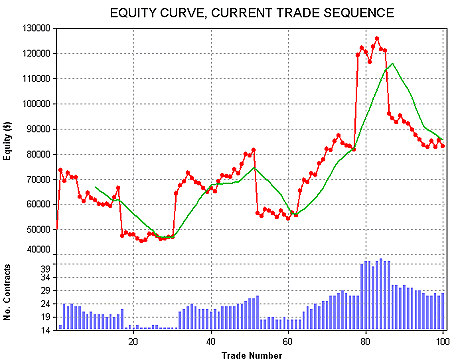Original equity curve and number of contracts prior to applying equity curve crossover rule. The equity curve is shown in red; a 10 period moving average of the equity curve is in green.

Notice that the equity curve tends to be mean reverting; that is, a series of wins is followed by a series of losses, which is followed by another series of wins, and so on. This is reflected in the number of contracts, which rises and falls dramatically in several places. A trading pattern of this type suggests that it may be better to stop trading when the equity curve crosses above its moving average (anticipating a reversion to losses) and resume trading when the equity curve falls below its moving average (anticipating a reversion to wins). Applying this approach for the trade series shown above produces the equity curve shown below.Equity curve and number of contracts with equity curve crossover rule applied. Trading is stopped on crossovers above the moving average; trading resumes on crossovers below the moving average. The equity curve is shown in red; a 10 period moving average of the equity curve is in green.

Not only does this change produce a gradually rising equity curve with more profit and a lower drawdown, but it does so with fewer trades. The blank areas in the bar chart below the equity curve indicates the trades that are skipped. The number of contracts for the trades that are taken tends to increase much more smoothly than before, without the dramatic rises and drops of the original curve.

A similar but more subtle effect could be achieved by increasing and/or decreasing the position size relative to the value determined from the dollar equity amount per contract (\$3000 in the example) on crossovers of the moving average.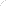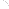Join Our Email List Email: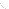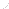For Email Marketing you can trust